DATA MINING
Desktop Survival Guide
by Graham Williams## Box Plot

A boxplot (, ) (also known as a box-and-whisker plot) provides a graphical overview of how data is distributed over the number line. Rattle's Box Plot displays a graphical representation of the textual summary of data. It is useful for quickly ascertaining the skewness of the distribution of the data. If we have identified a Target variable, then the boxplot will also show the distribution of the values of the variable partitioned by values of the target variable, as we illustrate for the variable Age where Adjusted has been chosen as the Target variable.

The boxplot (which here is shown with the Annotate option checked) shows the median (which is also called the second quartile or the 50th percentile) as the thicker line within the box (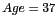over the whole population, as we can see from the Summary option's Summary check box). The top and bottom extents of the box (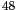and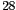respectively) identify the upper quartile (the third quartile or the 75th percentile) and the lower quartile (the first quartile and the 25th percentile). The extent of the box is known as the interquartile range (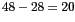). The dashed lines extend to the maximum and minimum data points that are no more than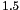times the interquartile range from the median. Outliers (points further thantimes the interquartile range from the median) are then individually plotted (at 79, 81, 82, 83, and 90). The mean (38.62) is also displayed as the asterisk.

The notches in the box, around the median, indicate a level of confidence about the value of the median for the population in general. It is useful in comparing the distributions, and in this instance it allows us to say that all three distributions being presented here have significantly different means. In particular we can state that the positive cases (where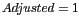) are older than the negative cases (where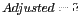).

We note that the annotated box plot (as enable by checking the Annotate check box) does not attempt to place the annotations in any particularly optimal location, except a little below the point being annotated. They may be a little difficult to read at times. The user is at liberty to correct thus through replicating the plotting steps from the log window, but modifying the offsets in the display of the annotations.